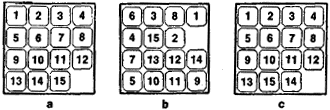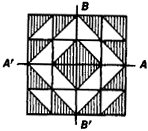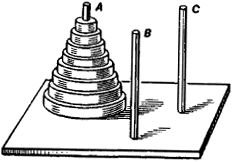# Mathematical Diversions and Games

The following article is from The Great Soviet Encyclopedia (1979). It might be outdated or ideologically biased.

## Mathematical Diversions and Games

Various problems and exercises of a diversionary nature that require resourcefulness, keenness of wit, originality of thought, and the ability to critically assess conditions or formulate the problem are usually called mathematical diversions. These include puzzles, problems involving changing one figure into another by cutting and transposition of parts, tricks based on computations, and mathematical games. Mathematical games include games that have to do with numbers, figures, and the like or those whose result can be predetermined by a preliminary theoretical analysis. With the development of mathematical game theory, the term “mathematical games” (in the sense of this article) is being used increasingly less. Below are two examples of games.

Example 1. Two players in turn draw no more than m (for example, five) articles from a pile containing n (for example, 35) articles. The one who draws the last articles is the winner. A result of game theory asserts that if n is not divisible by m 1, then the first player will necessarily win if he leaves his opponent after each turn a number of articles that is a multiple of m + 1 (in the example, a multiple of 6).

Example 2. This game involves one player. Fifteen numbered tiles are arranged randomly on a board with 16 cells. By first moving one tile and then another from any of the cells to an adjacent free cell, the player must arrange the tiles in the usual numerical order (see Figure 1, a). A theoretical analysis of the game, which has been known since 1879, shows that the problem can be solved only if the number of inversions (that is, the number of violations of the usual numerical order) in the initial arrangment of the numbered tiles is of the same parity as the number of the row (counting from the top) containing the free cell. To establish the number of inversions, it is necessary to calculate for each tile the quantity of tiles preceding it that have larger numbers and to add all these quantities; their sum will give the desired number of inversions. Here we say of a number that it precedes another number in the same row if it is to the left of it and that it precedes another number in the same column if it is above it. For example, in the arrangement given in Figure 1, b the number of inversions is even (38) and the free cell is in an even (second) row, that is, this arrangement can be reduced to the normal arrangement. On the other hand, the arrangement in Figure 1, c cannot be reduced to the normal arrangement since the number of inversions in it is odd (equal to 1: tile number 15 precedes tile number 14) and the free cell is in the fourth row (an even numbered row).Figure 1

A complete mathematical foundation also exists for such mathematical diversions as drawing figures in a continuous manner (unicursal curves), labyrinths, and combinatorial problems on a chessboard. A large group of mathematical diversions and games is associated with finding original and elegant solutions of problems having a practically inexhaustible number of solutions or even infinitely many solutions.

One such diversion is that of “forming parquets”; it consists in filling a plane with regularly alternating figures of the same type, (for example, with similar regular polygons) or with several given types of figures. If a “two-color square parquet” with axes of symmetry A′A and B′B (Figure 2) consists of 4n2 equal squares, each of which is divided by a diagonal into a white and a black half, then the number of different parquets is 4n2 (this number grows rapidly with increasing n).Figure 2

Among the diversions that have a very large, not yet exactly determined, number of solutions is Euler’s knight problem, which consists in moving a knight on a chessboard so that each square is traversed just once. Another such diversion is the problem of constructing multicelled magic squares. In such problems we usually wish to determine the number of solutions and develop methods that at once yield large groups of solutions. The mathematical content of a number of other mathematical diversions and games lies in determining the least number of operations required for achieving a stated objective. Such diversions include “ferry” and “arrangement” problems or games similar to the Hanoi Tower game, which consists in calculating the least number of steps necessary to transfer the disks from peg A (Figure 3) to peg C using peg B subject to the restriction that only one disc may be transferred at one time from any one peg to any other and that no larger disk may be placed above a smaller one.Figure 3

Mathematical diversions and games have drawn the attention of many major scientists, such as Leonardo of Pisa (13th century), N. Tartaglia (16th century), G. Cardano (16th century), G. Monge (second half of the 18th century and early 19th century), and L. Euler (18th century). Collections of mathematical diversions and games began appearing in the 17th century. Mathematical diversions and games, which help heighten interest in mathematics and develop quickness of wit and perseverance, are also used in the teaching process. In Russia, this was already reflected in L. F. Magnitskii’s Arithmetic (1703) and even in 17th-century mathematical manuscripts.

### REFERENCES

Ignat’ev, E. I. Vtsartsve smekalki Hiarifmetika dlia vsekh, 2nd ed., books 1-3. Moscow-Leningrad, 1924-25.
Kordemskii, B. A. Matematicheskaia smekalka, 8th ed. Moscow, 1965.
Perel’man, Ia. I. Zhivaia matematika, 9th ed. Moscow, 1970.
Perel’man, Ia. I. Zanimatel’naia arifmetika, 9th ed. Moscow, 1959.
Perel’man, Ia. I. Zanimatel’naia algebra, 12th ed. Moscow, 1970.
Perel’man, Ia. L Zanimatel’naia geometriia, 11th ed. Moscow, 1959.
Schubert, G. Matematicheskie razvlecheniia i igry. Odessa, 1911. (Translated from German.)
Ahrens, W. Matematicheskie igry. Leningrad-Moscow, 1924. (Translated from German.)
Gardner, M. Matematicheskie chudesa i tainy: Matematicheskie fokusy i golovolomki, 2nd ed. Moscow, 1967. (Translated from English.)
Gardner, M. Matematicheskie dosugi. Moscow, 1972. (Translated from English.)